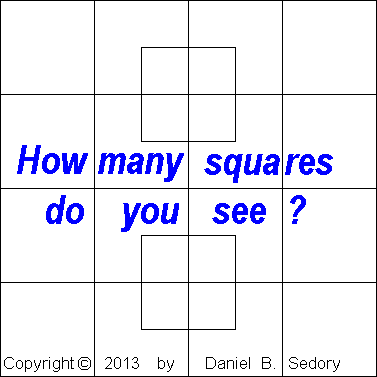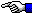# How many Squares in this Diagram:

## A Problem of PerspectiveThis problem depends on how perceptive you are at seeing what is right in front of you. The question is very simple: How many squares are there in this diagram? 8, 10, 16, 18, 24, 26 or more? If you counted only 8, 10 or 16, then you limited the number of squares by using a rule we never stated.

(Biggest hint ever: Any number of lines can be included within a square; as well as the BLUE lettering and format copyright we added to this particular diagram! Only if you imposed a defintion of square to mean a standard square that is completely empty inside, would the answer be 16; or only 10 if you also excluded our lettering. But we simply asked, "How many squares are in this diagram?")

For a complete answer to the question using animation, see our Total Number of the Squares Countdown Video.

The Starman.The Starman's Math Index